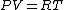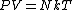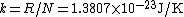# Boltzmann constant

Also found in: Dictionary, Thesaurus, Medical, Legal, Wikipedia.
Related to Boltzmann constant: Boltzmann distribution

## Boltzmann constant

A constant occurring in practically all statistical formulas and having a numerical value of 1.3807 × 10-23 joule/K. It is represented by the letter k. If the temperature T is measured from absolute zero, the quantity kT has the dimensions of an energy and is usually called the thermal energy. At 300 K (room temperature) kT = 0.0259 electronvolt.

The value of the Boltzmann constant may be determined from the ideal gas law. For 1 mole of an ideal gas Eq. (1a)

(1a)(1b)holds, where P is the pressure, V the volume, and R the universal gas constant. The value of R, 8.31 J/K mole, may be obtained from equation-of-state data. Statistical mechanics yields for the gas law Eq. (1b). Here N, the number of molecules in 1 mole, is called Avogadro's number and is equal to 6.02 × 1023 molecules/mole. Hence, comparing Eqs. (1a) and (1b), one obtains Eq. (2). (2)Almost any relation derived on the basis of the partition function or the Bose-Einstein, Fermi-Dirac, or Boltzmann distribution contains the Boltzmann constant. See Boltzmann statistics, Bose-Einstein statistics, Fermi-Dirac statistics, Kinetic theory of matter, Statistical mechanics

McGraw-Hill Concise Encyclopedia of Physics. © 2002 by The McGraw-Hill Companies, Inc.

## Boltzmann constant

(bohlts -măn, -mahn) Symbol: k . A constant given by the ratio of the molar gas constant to the Avogadro constant and equal to 1.380 658 × 10–23 joules per kelvin. It is the constant by which the mean kinetic energy of a gas particle can be related to its thermodynamic temperature.
Collins Dictionary of Astronomy © Market House Books Ltd, 2006
The following article is from The Great Soviet Encyclopedia (1979). It might be outdated or ideologically biased.

## Boltzmann Constant

one of the fundamental physical constants; equal to the ratio of the universal gas constant R to Avogadro’s number NA (the number of molecules in 1 mole of a substance): k = R/NA. It is named for L. Boltzmann. The Boltzmann constant enters into a number of the major relations of physics—the equation of the state of an ideal gas and the expression for the average energy of the thermal motion of particles (and thermal capacity itself). It also connects the entropy of a physical system with its thermodynamic probability.

The Boltzmann constant is k = (1.380622 ± 0.000059) x 10-23 joules per °K; this value corresponds to the most accurate data (as of 1969) on the constants R and NA. The value of the Boltzmann constant can be determined directly, for example, by the experimental verification of the laws of radiation. [3–1572–1; updated]

The Great Soviet Encyclopedia, 3rd Edition (1970-1979). © 2010 The Gale Group, Inc. All rights reserved.

## Boltzmann constant

[′bōlts·mən ‚kän·stənt]
(statistical mechanics)
The ratio of the universal gas constant to the Avogadro number.
McGraw-Hill Dictionary of Scientific & Technical Terms, 6E, Copyright © 2003 by The McGraw-Hill Companies, Inc.
References in periodicals archive ?
This results in a relative measurement uncertainty for the cross-sectional area of 0.7 x [10.sup.-6], which may well contribute to decreasing the measurement uncertainty for the Boltzmann constant as a whole, Mahr Federal says.
When the resistance of the thermal noise source is expressed in terms of the conventional value of the von Klitzing constant [R.sub.K-90], the measured ratio <[V.sup.2.sub.R]>/<[V.sup.2.sub.JJ]> will be proportional to k/h, the ratio of the Boltzmann constant to the Planck constant.
where [[sigma].sub.0] is the preexponential factor, [[epsilon].sub.a(ac)] is the activation energy, and [k.sub.B] is the Boltzmann constant.
where T is the absolute temperature, k is the Boltzmann constant, [gamma] is the thermal noise coefficient and is typically 2/3 for the MOSFETs.
As can be seen from Appendix B, the New SI is based on the set of seven reference constants [DELTA]v[([.sup.133]Cs).sub.hfs], the ground state hyperfine splitting frequency of the cesium 133 atom; c, the speed of light in vacuum; h, the Planck constant; e, the elementary charge (charge of a proton); k, the Boltzmann constant; [N.sub.A], the Avogadro constant; and [K.sub.cd], the luminous efficacy of monochromatic radiation of frequency 540 x [10.sup.12] Hz.
where + and - signs correspond to hole and electron type of conductivity, [E.sub.s] is the activation energy for TEP, [alpha] is a constant and [alpha] < 1 suggests that the conductivity is small polaron hopping, whereas for [alpha] > 2, conductivity is due to large polarons, and k is the Boltzmann constant. The [alpha] values for the first and second regions were found to be 1.47 and 0.85, respectively.
That the Boltzmann constant is shows with k the surface potential (FS) and temperature (T) the valence of the electrolyte (Z) the surface charge density(s) dielectric constant (e) and the electrolyte concentration(c) in the solution.
Here, [[kappa].sub.B] is Boltzmann constant, T is the absolute temperature of the solution, [eta] is the viscosity of the solvent medium, and d is the calculated hydrodynamic diameter of the nanoparticles.
where [D.sub.0] is the diffusion constant, [E.sub.a] is the activation energy, and k is the Boltzmann constant (1.3807 x [10.sup.-23] J/K).
where [[f.sub.0](E)= {1 + exp[(E-[E.sub.F])/[k.sub.B]T]}.sup.-1] is the Fermi-Dirac distribution function, [E.sub.F] is the Fermi energy in eV, [[rho].sub.c](E) and [[rho].sub.v](E) are, respectively, the electron density of states for the conduction band and the hole density of states for the valence band, [k.sub.B] is the Boltzmann constant, and T is the temperature in kelvins.

Site: Follow: Share:
Open / Close